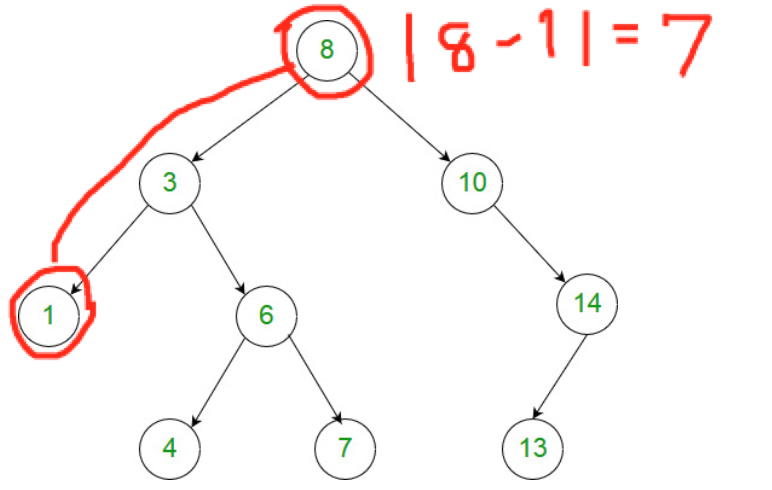# 1026. Maximum Difference Between Node and Ancestor

## Problem Setting¶

Given the root of a binary tree, find the maximum value V for which there exists different nodes A and B where V = |A.val - B.val| and A is an ancestor of B.

(A node A is an ancestor of B if either: any child of A is equal to B, or any child of A is an ancestor of B.)

Note:

• The number of nodes in the tree is between 2 and 5000.
• Each node will have value between 0 and 100000.

### Example 1¶

Input: [8,3,10,1,6,null,14,null,null,4,7,13]Output: 7

Explanation:

We have various ancestor-node differences, some of which are given below : |8 - 3| = 5 |3 - 7| = 4 |8 - 1| = 7 |10 - 13| = 3 Among all possible differences, the maximum value of 7 is obtained by |8 - 1| = 7.

## Solution¶

### Solution 1 from superluminal¶

In :
class Solution(object):
def maxAncestorDiff(self, root):
"""
:type root: TreeNode
:rtype: int
"""
r = 
def f(node, a, b):
if not node: return
r = max(r, abs(node.val-a), abs(node.val-b))
a = min(a, node.val)
b = max(b, node.val)
f(node.left, a, b)
f(node.right, a, b)
f(root, root.val, root.val)
return r


#### Step-by-step¶

In :
# Definition for a binary tree node.
class TreeNode(object):
def __init__(self, x):
self.val = x
self.left = None
self.right = None

class Solution(object):
def maxAncestorDiff(self, root):
"""
:type root: TreeNode
:rtype: int
"""
r = 
def f(node, a, b):
if not node: return
r = max(r, abs(node.val-a), abs(node.val-b))
a = min(a, node.val)
b = max(b, node.val)
f(node.left, a, b)
f(node.right, a, b)
f(root, root.val, root.val)
return r


#### Execute the entire class¶

In :
# Execute the entire class
root = TreeNode(1)
root.left = TreeNode(3)
root.left.left = TreeNode(5)
root.right = TreeNode(2)

s = Solution()
s.maxAncestorDiff(root)

Out:
4

#### Execute recursive function f¶

a and b stores the minimum and maximum value across calculation. r is the result value, which is compared with the absolute distance between the value at the current node and the minimum or maximum in the ancestor path. Then, recursively this function f is called and stores the maximum of absolute distance in the ancestor path.

I noticed that r is actually not necessarily to be a list. It can be just a value.

In :
def f(node, a, b):
if not node: return
print('Processing at {}'.format(node.val))
print('Compare {}, {}, {}'.format(r, abs(node.val-a), abs(node.val-b)))
r = max(r, abs(node.val-a), abs(node.val-b))
print('Updated the maximum distance to {}'.format(r))
a = min(a, node.val)
b = max(b, node.val)
f(node.left, a, b)
f(node.right, a, b)


The example of f execution as below returns 4 between the root for 1 and the left bottom node for 5.

(root)
(1)
/ \
3   2
/
(5)
In :
r = 
f(root, root.val, root.val)

Processing at 1
Compare 0, 0, 0
Updated the maximum distance to 0
Processing at 3
Compare 0, 2, 2
Updated the maximum distance to 2
Processing at 5
Compare 2, 4, 2
Updated the maximum distance to 4
Processing at 2
Compare 4, 1, 1
Updated the maximum distance to 4

In :
# Result is 4 between node 1 and 5
r

Out:


This is essentially the same as the above. The key is to create the recursive function for depth first search to keep minimum and maximum values across ancestor path.

In [ ]:
# Definition for a binary tree node.
# class TreeNode:
#     def __init__(self, x):
#         self.val = x
#         self.left = None
#         self.right = None

class Solution:
def maxAncestorDiff(self, root: TreeNode) -> int:
def dfs(node, mn=math.inf, mx=-math.inf):
if node:
mn = min(mn, node.val)
mx = max(mx, node.val)
dfs(node.left, mn, mx)
dfs(node.right, mn, mx)
else:
self.ans = max(self.ans, mx - mn)
self.ans = 0
dfs(root)
return self.ans